# Measurement of Optical Activity

## Optical Activity

– Optical activity is one of imortant physcial properties of liqiuds

– A beam of ordinary light consists of electromagnetic waves oscillating in many planes.

– When passed through a polarizer (e.g., a Polaroid lens), only waves oscillating in a single plane pass through.

The emerging beam of light having oscillations in a single plane is said to be planepolarized.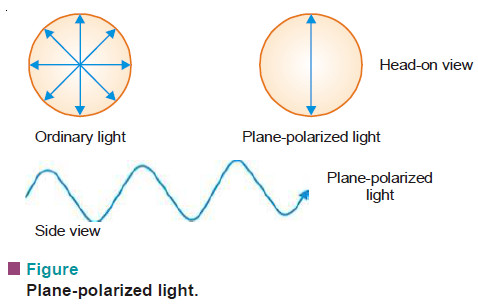– When plane-polarized light is passed through certain organic compounds, the plane of polarized light is rotated.

A compound that can rotate the plane of polarized light is called optically active. This property of a compound is called optical activity.

– A compound which rotates the plane-polarized light to the left (anticlockwise), is said to be levorotatory.

– A compound that rotates the plane-polarized light to the right (clockwise), is said to be dextrorotatory.

– By convention, rotation to the left is given a minus sign (–) and rotation to the right is given a plus sign (+).

– For example, (–)-lactic acid is levorotatory and (+)-lactic acid is dextrorotatory.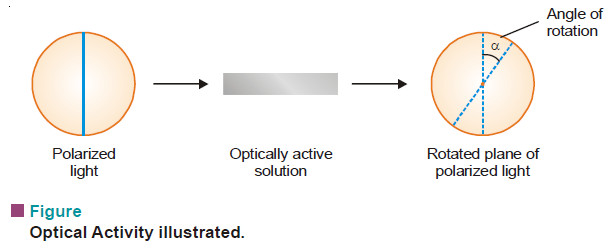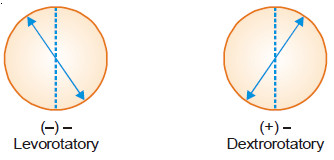## Specific Rotation

– The rotation of plane-polarized light is an intrinsic property of optically active molecules.

– When a polarized beam of light is passed through the solution of an optically active compound, its plane is rotated through an angle (α) (angle of rotation).

– This rotation depends on the number of optically active molecules encountered. Therefore, α is proportional to both the concentration and the length of the sample solution.

– The specific rotation which is characteristic of an optically active substance, is expressed as: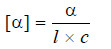where:

[α] = specific rotation in degrees
α = observed angle of rotation in degrees
l = length of the sample solution in decimeters (dm; 10 cm)
c = concentration of the sample solution in g/ml

– Thus fromthe previous equation, the specific rotation can be defined as the observed angle of rotation at a concentration of 1 g/ml and path length of 1 dm.

– Conventionally, a specific rotation is reported as [α]tD , where (t) stands for temperature and (D) for D-line of sodium used for determination.

## Measurement of Optical Activity

– Optical activity is measured with the help of an instrument known as polarimeter .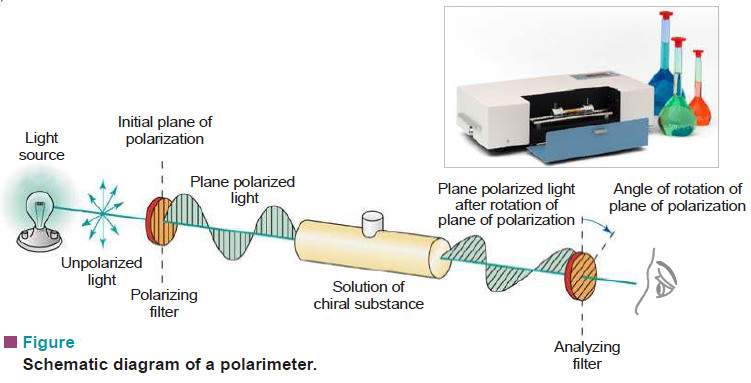– This is basically a system of polarizers with a sample tube placed in between.

– First, an optically inactive medium (air or solvent) fills the sample tubes and polarized sodium light emerging from the polarizer passes through it.

– The analyzer is then turned to establish a dark field. This gives a zero reading on the circular scale around the analyzer.

– Then the solution of the given optically active compound is placed in the sample tube.

– The plane of polarized light passing through it is rotated. The analyzer is turned to re-establish the dark field.

– The angle of rotation (α) is then noted in degrees on the circular scale.

– The specific rotation is calculated using the expression: